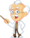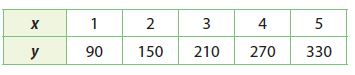Homework Explained - Math Practice 101Dear guest, you are not a registered member. As a guest, you only have read-only access to our books, tests and other practice materials.

As a registered member you can:

 Question 10 Analyze Relationships A proportional relationship is a linear relationship because the rate of change is constant (and equal to the constant of proportionality). What is required of a proportional relationship that is not required of a general linear relationship? Type below:(show solution) Question 11 Communicate Mathematical Ideas Explain how you can identify a linear non-proportional relationship from a table, a graph, and an equation. Type below:(show solution) H.O.T.     Focus on Higher Order Thinking Question 12 Critique Reasoning George observes that for every increase of 1 in the value of x, there is an increase of 60 in the corresponding value of y. He claims that the relationship represented by the table is proportional. Critique George’s reasoning.Type below:(show solution) Question 13 Make a Conjecture Two parallel lines are graphed on a coordinate plane. How many of the lines could represent proportional relationships? Explain. Type below:(show solution)